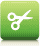2016

18Math（算数）对象的作用是：执行常见的算数任务。round()

random()

max()

min()

## Math 对象

Math（算数）对象的作用是：执行普通的算数任务。

Math 对象提供多种算数值类型和函数。无需在使用这个对象之前对它进行定义。

var x=Math.PI;
var y=Math.sqrt(16);

## 算数值

JavaScript 提供 8 种可被 Math 对象访问的算数值：

Math.E
Math.PI
Math.SQRT2
Math.SQRT1_2
Math.LN2
Math.LN10
Math.LOG2E
Math.LOG10E

## 算数方法

document.write(Math.round(4.7));

5

document.write(Math.random());

document.write(Math.floor(Math.random()*11));